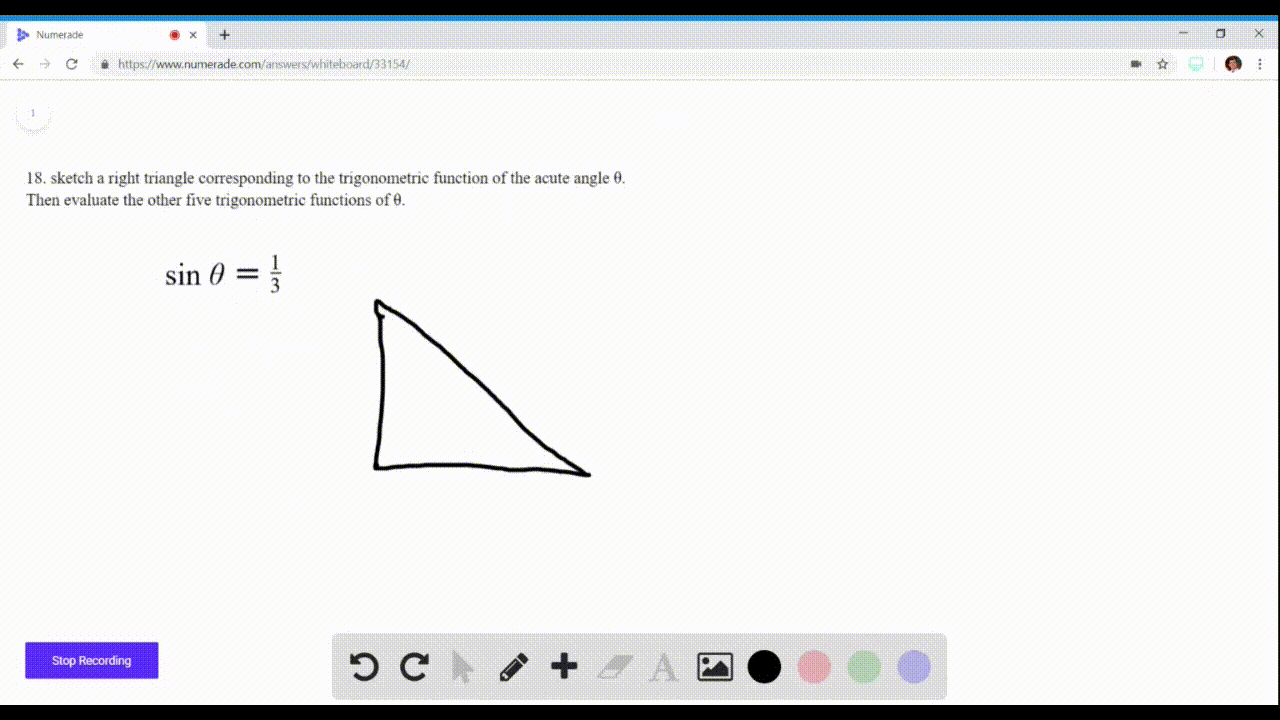Enroll in one of our FREE online STEM summer camps. Space is limited so join now!View Summer Courses### Evaluating Trigonometric Functions In Exercises $… 02:42University of California, Berkeley Need more help? Fill out this quick form to get professional live tutoring. Get live tutoring Problem 17 Evaluating Trigonometric Functions In Exercises$17-20$, sketch a right triangle corresponding to the trigonometric function of the acute angle$\theta$. Then evaluate the other five trigonometric functions of$\theta\$ .
$$\sin \theta=\frac{1}{2}$$

## Discussion

You must be signed in to discuss.

## Video Transcript

Okay, Well, given that sine of data is, he could feel 1/2. So drawing out that triangle, you know that opposite is one. And hi, pop music too. So now we just need to find this term on the bottom so we can use our people agree and dim. You know that R squared is equal to x squared. Plus y quite you know that. Why is one that's one squared plus x squared? Because our word, which is two squared, which is what? So we have four minutes one x squared, which means that X is equal to the square root of three. Okay, so this is clearly a defeat so that we can find a other angles. So we know that coastline of data. The people here, Jason over. Hi, Panis. Tangents of data he could to opposite over adjacent anything. My pockets are not over opposite Beacon of data is about a typical of co sign that's two over square root of B. And speaking of data is difficult off sign, which is to over one